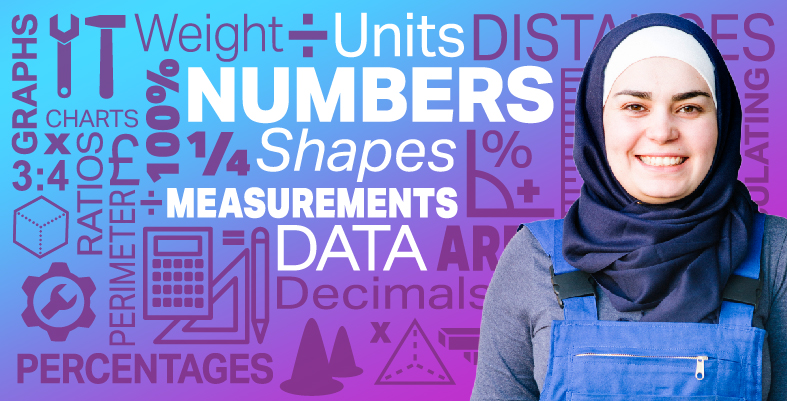Everyday maths for Construction and Engineering 1

Start this free course now. Just create an account and sign in. Enrol and complete the course for a free statement of participation or digital badge if available.

Free course

# 12 Session 1 summary

You have now completed Session 1, ‘Working with numbers’. If you have identified any areas that you need to work on, please ensure you refer back to this section of the course and retry the activities.

You should now be able to:

• understand and use whole numbers, and understand negative numbers in practical contexts
• add, subtract, multiply and divide whole numbers using a range of strategies
• understand and use equivalences between common fractions, decimals and percentages
• add and subtract decimals up to two decimal places
• solve simple problems involving ratio, where one number is a multiple of the other
• use simple formulas expressed in words for one- or two-step operations.

All of the skills above will help you with tasks in everyday life. Whether you are at home or at work, number skills are an essential skill to have.

You are now ready to move on to Session 2, ‘Units of measure’.

FSM_SSC_1